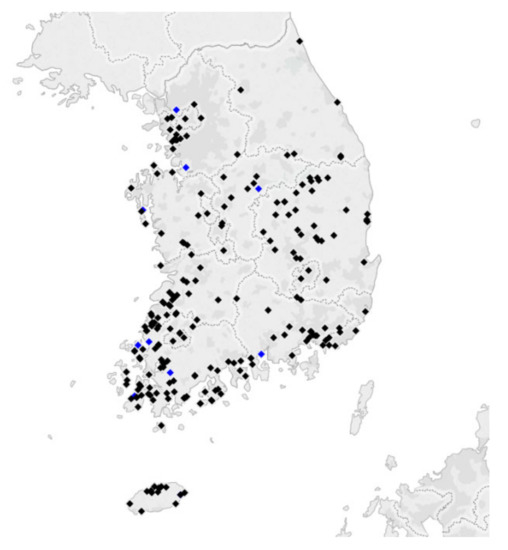Previous Article in Journal
Low-Carbon Impact of Urban Rail Transit Based on Passenger Demand Forecast in Baoji
Previous Article in Special Issue
Effect of RF Power on the Properties of Sputtered-CuS Thin Films for Photovoltaic Applications
Open AccessArticle

# Linear-Gompertz Model-Based Regression of Photovoltaic Power Generation by Satellite Imagery-Based Solar Irradiance

byAlba Vilanova 1,2,Bo-Young Kim 1,Chang Ki Kim 1 andHyun-Goo Kim 1,*1
New-Renewable Energy Resource & Policy Center, Korea Institute of Energy Research, Daejeon 34129, Korea
2
Higher Polytechnic School, University of Lleida, 25001 Lleida, Spain
*
Author to whom correspondence should be addressed.
Energies 2020, 13(4), 781; https://doi.org/10.3390/en13040781
Received: 31 December 2019 / Revised: 4 February 2020 / Accepted: 8 February 2020 / Published: 11 February 2020
A simple yet accurate photovoltaic (PV) performance curve as a function of satellite-based solar irradiation is necessary to develop a PV power forecasting model that can cover all of South Korea, where more than 35,000 PV power plants are currently in operation. In order to express the nonlinear power output of the PV module with respect to the hourly global horizontal irradiance derived from satellite images, this study employed the Gompertz model, which is composed of three parameters and the sigmoid equation. The nonphysical behavior of the Gompertz model within the low solar irradiation range was corrected by combining a linear equation with the same gradient at the conjoint point. The overall fitness of Linear-Gompertz regression to the 242 PV power plants representing the country was R2 = 0.85 and nRMSE = 0.09. The Gompertz model coefficients showed normal distributions and equivariance of standard deviations of less than 15% by year and by season. Therefore, it can be conjectured that the Linear-Gompertz model represents the whole country’s PV system performance curve. In addition, the Gompertz coefficient C, which controls the growth rate of the curve, showed a strong correlation with the capacity factor, such that the regression equation for the capacity factor could be derived as a function of the three Gompertz model coefficients with a fitness of R2 = 0.88. View Full-Text
Show FiguresFigure 1

MDPI and ACS Style

Vilanova, A.; Kim, B.-Y.; Kim, C.K.; Kim, H.-G. Linear-Gompertz Model-Based Regression of Photovoltaic Power Generation by Satellite Imagery-Based Solar Irradiance. Energies 2020, 13, 781.